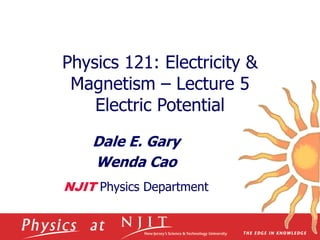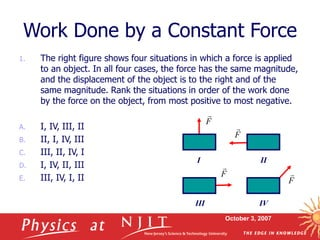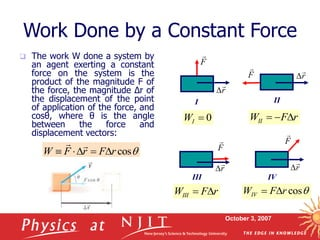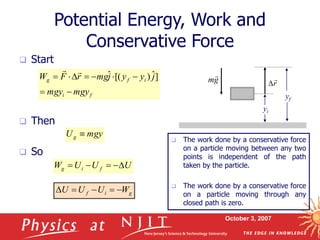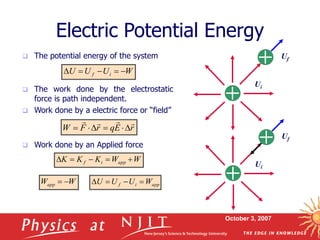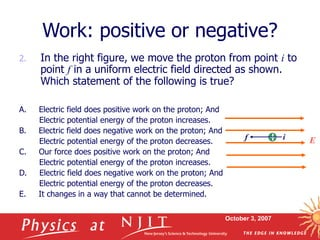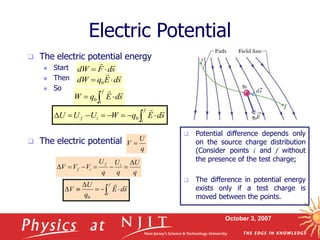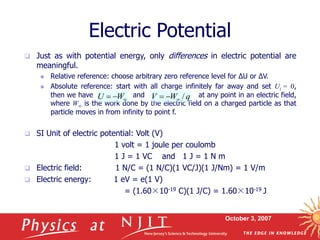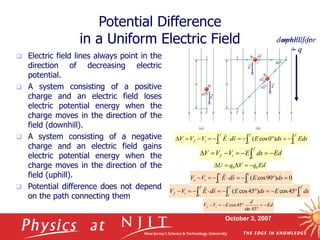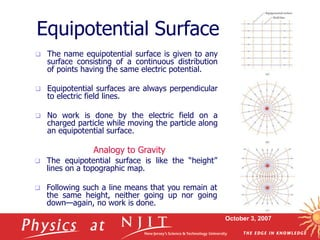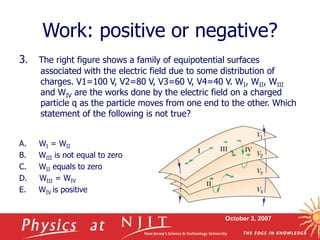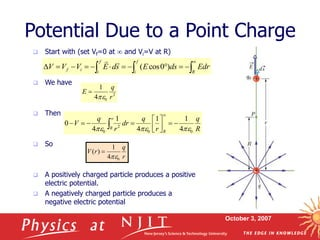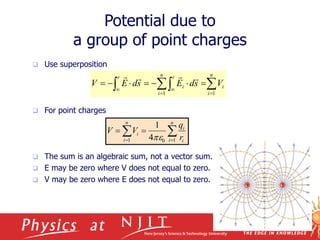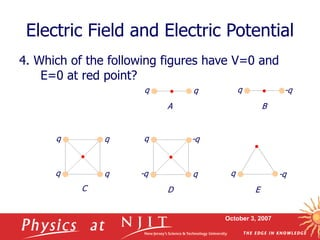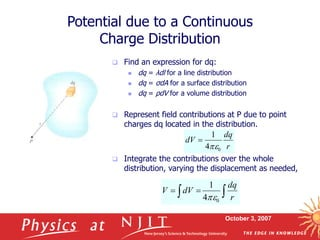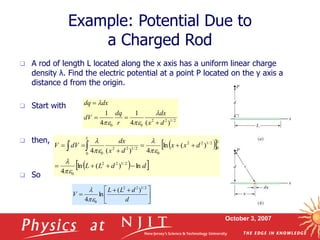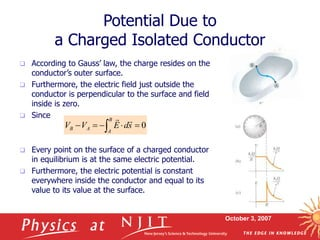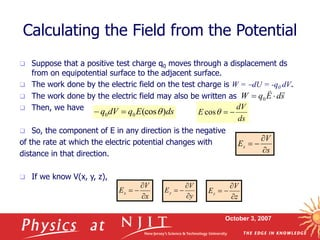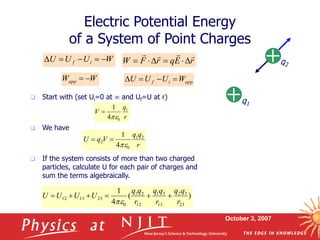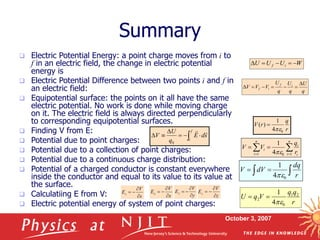1 of 20

## RecommendedElectrostatics 3Shwetha Inspiringelectrostatics_3.pptInfiniteGaming12Electrostatics Class 12- Part 3Self-employedphysics121_lecture03.pptJpBesaElectrostatics - 203PHYSSabar D HutagalungJEE Main 12 Sample ebookMiso Study

### physics121_lecture05.ppt

1. Physics 121: Electricity & Magnetism – Lecture 5 Electric Potential Dale E. Gary Wenda Cao NJIT Physics Department
2. October 3, 2007 Work Done by a Constant Force 1. The right figure shows four situations in which a force is applied to an object. In all four cases, the force has the same magnitude, and the displacement of the object is to the right and of the same magnitude. Rank the situations in order of the work done by the force on the object, from most positive to most negative. A. I, IV, III, II B. II, I, IV, III C. III, II, IV, I D. I, IV, II, III E. III, IV, I, II F  I F  II F  III F  IV
3. October 3, 2007 Work Done by a Constant Force  The work W done a system by an agent exerting a constant force on the system is the product of the magnitude F of the force, the magnitude Δr of the displacement of the point of application of the force, and cosθ, where θ is the angle between the force and displacement vectors:  cos r F r F W        F  II F  III r   F  I r   F  IV r   r   0  I W  cos r F WIV   r F WIII   r F WII   
4. October 3, 2007 Potential Energy, Work and Conservative Force  Start  Then  So f i i f g mgy mgy j y y j mg r F W          ] ˆ ) [( ˆ   mgy Ug  U U U W f i g      g i f W U U U       The work done by a conservative force on a particle moving between any two points is independent of the path taken by the particle.  The work done by a conservative force on a particle moving through any closed path is zero. yf yi r   g m 
5. October 3, 2007  The potential energy of the system  The work done by the electrostatic force is path independent.  Work done by a electric force or “field”  Work done by an Applied force Electric Potential Energy Ui Uf W U U U i f      r E q r F W           Ui Uf W W K K K app i f      W Wapp   app i f W U U U    
6. October 3, 2007 2. In the right figure, we move the proton from point i to point f in a uniform electric field directed as shown. Which statement of the following is true? A. Electric field does positive work on the proton; And Electric potential energy of the proton increases. B. Electric field does negative work on the proton; And Electric potential energy of the proton decreases. C. Our force does positive work on the proton; And Electric potential energy of the proton increases. D. Electric field does negative work on the proton; And Electric potential energy of the proton decreases. E. It changes in a way that cannot be determined. Work: positive or negative? E i f
7. October 3, 2007  The electric potential energy  Start  Then  So  The electric potential Electric Potential q U V  q U q U q U V V V i f i f        s d F dW     s d E q dW     0 s d E q W f i      0          f i i f s d E q W U U U   0        f i s d E q U V   0  Potential difference depends only on the source charge distribution (Consider points i and f without the presence of the test charge;  The difference in potential energy exists only if a test charge is moved between the points.
8. October 3, 2007  Just as with potential energy, only differences in electric potential are meaningful.  Relative reference: choose arbitrary zero reference level for ΔU or ΔV.  Absolute reference: start with all charge infinitely far away and set Ui = 0, then we have and at any point in an electric field, where W is the work done by the electric field on a charged particle as that particle moves in from infinity to point f.  SI Unit of electric potential: Volt (V) 1 volt = 1 joule per coulomb 1 J = 1 VC and 1 J = 1 N m  Electric field: 1 N/C = (1 N/C)(1 VC/J)(1 J/Nm) = 1 V/m  Electric energy: 1 eV = e(1 V) = (1.60×10-19 C)(1 J/C) = 1.60×10-19 J Electric Potential    W U q W V /   
9. October 3, 2007 uphill for  q  Electric field lines always point in the direction of decreasing electric potential.  A system consisting of a positive charge and an electric field loses electric potential energy when the charge moves in the direction of the field (downhill).  A system consisting of a negative charge and an electric field gains electric potential energy when the charge moves in the direction of the field (uphill).  Potential difference does not depend on the path connecting them Potential Difference in a Uniform Electric Field Ed ds E V V V f i i f                       f i f i f i i f Eds ds E s d E V V V ) 0 cos (                f c f c f c i f ds E ds E s d E V V 45 cos ) 45 cos (             c i c i i c ds E s d E V V 0 ) 90 cos (   Ed q V q U 0 0      Ed d E V V i f        45 sin 45 cos downhill for + q
10. October 3, 2007 Equipotential Surface  The name equipotential surface is given to any surface consisting of a continuous distribution of points having the same electric potential.  Equipotential surfaces are always perpendicular to electric field lines.  No work is done by the electric field on a charged particle while moving the particle along an equipotential surface.  The equipotential surface is like the “height” lines on a topographic map.  Following such a line means that you remain at the same height, neither going up nor going down—again, no work is done. Analogy to Gravity
11. October 3, 2007 3. The right figure shows a family of equipotential surfaces associated with the electric field due to some distribution of charges. V1=100 V, V2=80 V, V3=60 V, V4=40 V. WI, WII, WIII and WIV are the works done by the electric field on a charged particle q as the particle moves from one end to the other. Which statement of the following is not true? A. WI = WII B. WIII is not equal to zero C. WII equals to zero D. WIII = WIV E. WIV is positive Work: positive or negative?
12. October 3, 2007 Potential Due to a Point Charge  Start with (set Vf=0 at  and Vi=V at R)  We have  Then  So  A positively charged particle produces a positive electric potential.  A negatively charged particle produces a negative electric potential 2 0 4 1 r q E   2 0 4 1 r q E                  f i R f i i f Edr ds E s d E V V V ) 0 cos (   r q r V 0 4 1 ) (   R q r q dr r q V R R 0 0 2 0 4 1 1 4 1 4 0                  
13. October 3, 2007 Potential due to a group of point charges  Use superposition  For point charges  The sum is an algebraic sum, not a vector sum.  E may be zero where V does not equal to zero.  V may be zero where E does not equal to zero.               n i i n i r i r V s d E s d E V 1 1           n i i i n i i r q V V 1 0 1 4 1  q q q -q
14. October 3, 2007 4. Which of the following figures have V=0 and E=0 at red point? Electric Field and Electric Potential A q -q B q q q q q q C D q E -q q -q -q q
15. October 3, 2007  Find an expression for dq:  dq = λdl for a line distribution  dq = σdA for a surface distribution  dq = ρdV for a volume distribution  Represent field contributions at P due to point charges dq located in the distribution.  Integrate the contributions over the whole distribution, varying the displacement as needed, Potential due to a Continuous Charge Distribution r dq dV 0 4 1       r dq dV V 0 4 1 
16. October 3, 2007  A rod of length L located along the x axis has a uniform linear charge density λ. Find the electric potential at a point P located on the y axis a distance d from the origin.  Start with  then,  So Example: Potential Due to a Charged Rod 2 / 1 2 2 0 0 ) ( 4 1 4 1 d x dx r dq dV dx dq                 d d L L d x x d x dx dV V L L ln ) ( ln 4 ) ( ln 4 ) ( 4 2 / 1 2 2 0 0 2 / 1 2 2 0 0 2 / 1 2 2 0                            d d L L V 2 / 1 2 2 0 ) ( ln 4 
17. October 3, 2007  According to Gauss’ law, the charge resides on the conductor’s outer surface.  Furthermore, the electric field just outside the conductor is perpendicular to the surface and field inside is zero.  Since  Every point on the surface of a charged conductor in equilibrium is at the same electric potential.  Furthermore, the electric potential is constant everywhere inside the conductor and equal to its value to its value at the surface. Potential Due to a Charged Isolated Conductor 0       B A A B s d E V V  
18. October 3, 2007 s d E q W     0  Suppose that a positive test charge q0 moves through a displacement ds from on equipotential surface to the adjacent surface.  The work done by the electric field on the test charge is W = dU = -q0 dV.  The work done by the electric field may also be written as  Then, we have  So, the component of E in any direction is the negative of the rate at which the electric potential changes with distance in that direction.  If we know V(x, y, z), Calculating the Field from the Potential z V Ez     x V Ex     ds E q dV q ) (cos 0 0    ds dV E    cos s V Es     y V Ey    
19. October 3, 2007 Electric Potential Energy of a System of Point Charges  Start with (set Ui=0 at  and Uf=U at r)  We have  If the system consists of more than two charged particles, calculate U for each pair of charges and sum the terms algebraically. r q V 1 0 4 1   r q q V q U 2 1 0 2 4 1    ) ( 4 1 23 3 2 13 3 1 12 2 1 0 23 13 12 r q q r q q r q q U U U U        W Wapp   app i f W U U U     W U U U i f      r E q r F W           q1 q2
20. October 3, 2007 Summary  Electric Potential Energy: a point charge moves from i to f in an electric field, the change in electric potential energy is  Electric Potential Difference between two points i and f in an electric field:  Equipotential surface: the points on it all have the same electric potential. No work is done while moving charge on it. The electric field is always directed perpendicularly to corresponding equipotential surfaces.  Finding V from E:  Potential due to point charges:  Potential due to a collection of point charges:  Potential due to a continuous charge distribution:  Potential of a charged conductor is constant everywhere inside the conductor and equal to its value to its value at the surface.  Calculatiing E from V:  Electric potential energy of system of point charges: W U U U i f      q U q U q U V V V i f i f        r q r V 0 4 1 ) (         n i i i n i i r q V V 1 0 1 4 1      r dq dV V 0 4 1  s V Es     z V Ez     x V Ex     y V Ey     r q q V q U 2 1 0 2 4 1           f i s d E q U V   0